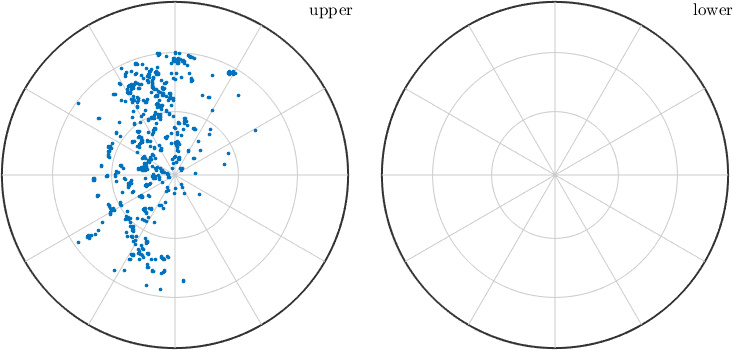Vector Operations edit page

In MTEX, one can calculate with three dimensional directions as with ordinary numbers, i.e. we can use the predefined vectors vector3d.X, vector3d.Y, and vector3d.Z and set

Moreover, all basic vector operations as "+", "-", "*", inner product, cross product are implemented in MTEX.

Besides the standard linear algebra operations, there are also the following functions available in MTEX.

 angle between two specimen directions inner product cross product length of the specimen directions normalize length to 1 sum over all specimen directions in v mean over all specimen directions in v conversion to spherical coordinates

A simple example for applying the norm function is to normalize a set of specimen directions

## Lists of vectors

As any other MTEX variable you can combine several vectors to a list of vectors. Additionally, all the operators operations mentioned before will work elementwise on a list of vectors. See Working with lists on how to manipulate lists in Matlab.

Using the brackets v = [v1,v2] two lists of vectors can be joined to a single list. Now each single vector is accesable via v(1) and v(2).

When calculating with concatenated specimen directions all operations are performed componentwise for each specimen direction.

A list of vectors can be indexed directly by specifying the ids of the vectors one is interested in, e.g.

gives the first 5 vectors from the list, or by logical indexing. The following command plots all vectors with an polar angle smaller then 60 degree